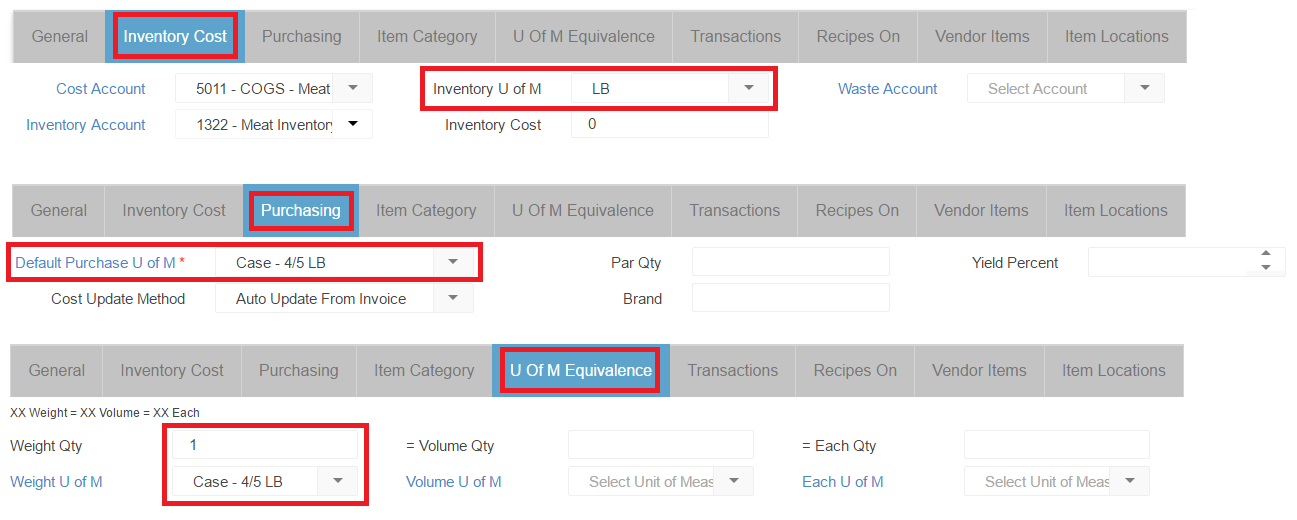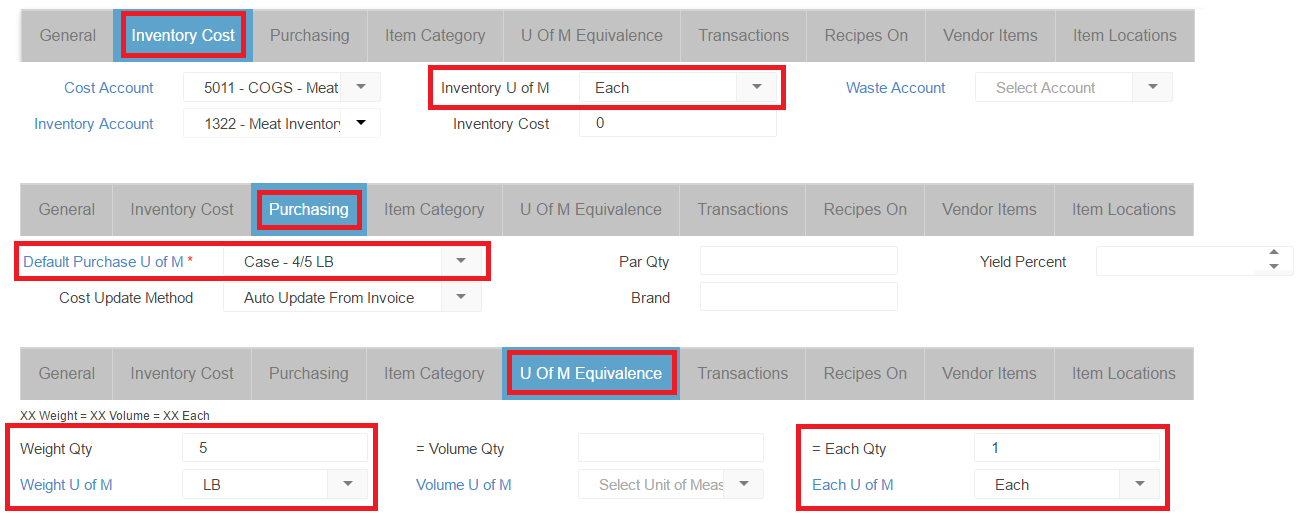In Restaurant365, there are three Measure Types for Units of Measure (U of M):

• Weight (Ounces)
• Volume (Fluid Ounces)
• Each

For each Purchased Item in the system, you will need to identify two units of measure:

• Purchased (Default Purchase U of M)
• Inventoried (Inventory U of M)

If the Purchased U of M and Inventoried U of M disagree in their Measure Type (Weight, Volume or Each), then an equivalence must be built so that Restaurant365 can properly identify item costs for Recipes, Inventory Counts, etc.

It is important to note that this tab will prepopulate with the Inventory UofM or Purchase UofM depending on which is selected first. If any information is cleared out of this tab, this will cause issues within the system.

Determine if U of M Equivalence is needed

To determine if a different Measure Type exists between the Purchasing U of M and Inventory U of M, review the Purchasing tab and Inventory Cost tab to identify the U of M's on the item (for Recipes, you would review the Recipe tab).

• Note: Measure Types are the same (Weight = Weight, Volume = Volume, Each = Each).

Scenario 1:

For items where the Inventory Measure Type and Purchase Measure Type are the same, no equivalence is required. The U of M Equivalence tab would not need to be updated. See the example screenshots below.

• Note: the Purchase U of M appears in the U of M Equivalence tab in it's respective section (in this example, Case - 4/5 LB is a Weight U of M, therefore it appears in the Weight Qty and Weight U of M fields).  As long as no values are placed in the Volume or Each section fields, this will not adversely affect our item or it's U of M Equivalence in the system.

Example 1

• Inventory U of M = LB (Weight)
• Purchase U of M = Case - 4/5 LB (Weight)
• Weight = WeightScenario 2:

Measure Types are different (Weight ≠ Volume, Weight ≠ Each, Volume ≠ Weight, Volume ≠ Each, Each ≠ Weight, Each ≠ Volume)

For Items where the Inventory U of M Measure Type and Purchasing U of M Measure Type are not the same, an equivalence will need to be identified.  The U of M Equivalence tab will need to be updated.  See the example screenshots below.

Example 2

• Inventory U of M = Each (Each)
• Purchase U of M = Case - 4/5 LB (Weight)
• Each ≠ Weight

In this example the Purchase U of M has 4 packs weighing 5 LBS, for a total of 20 LBS.  The Inventory U of M is set to Each, meaning the Item is being counted by the individual 5 LB pack.  On the U of M Equivalence tab the Weight Qty is set to 5, and the Weight U of M is set to LB.  The Each Qty is set to 1, and the Each U of M is set to Each.